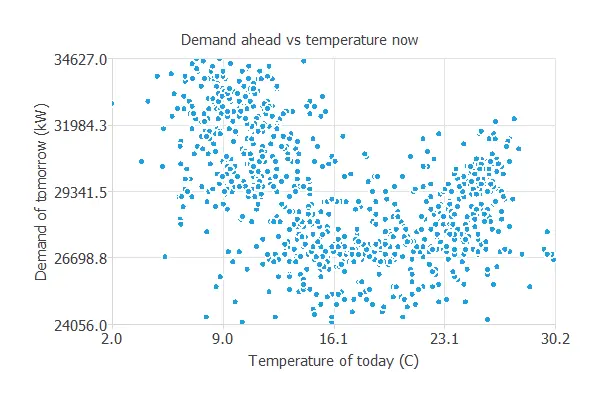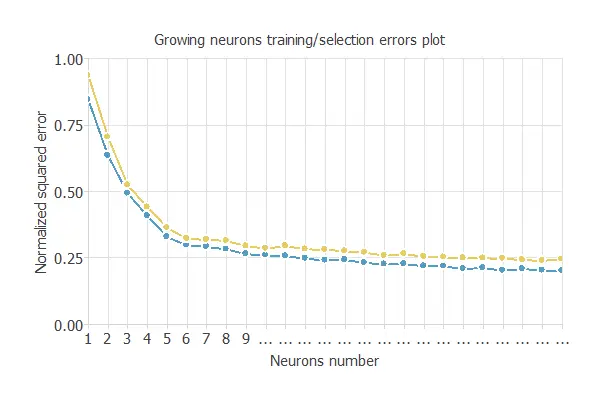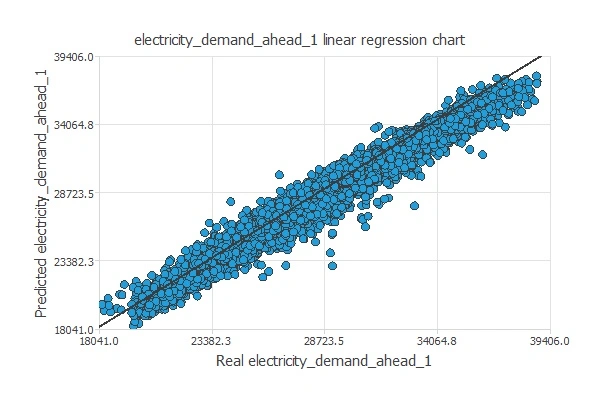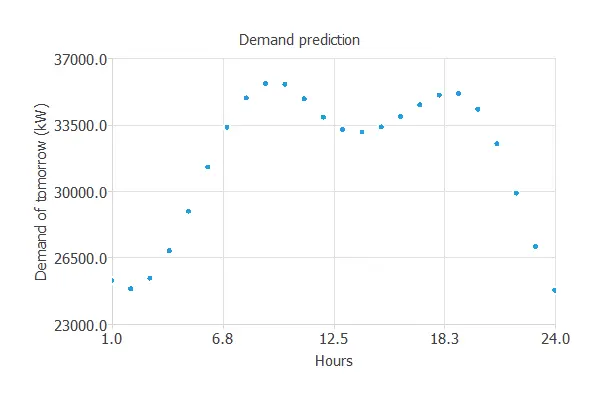Forecasting power demand plays an essential role in the electric industry. Indeed, it provides the basis for decision-making in power system planning and operation.

Electrical companies use various methods for predicting electricity demand. These are applied to short-term, medium-term, or long-term forecasting.

However, electricity use arises from complex interactions between meteorological and socio-economic factors. Standard forecasting techniques are insufficient in such a dynamic environment, and more sophisticated methods are needed.

The objective is to effectively untangle all the factors that lead to demand change and determine the underlying causes. This post explains how machine learning can help predict energy demand.

### Contents

To illustrate this process, we build a neural network for predicting the demand for electricity using a real data set taken from Kaggle, one of the most important repositories of data science.

## 1. Data set

A good model for predicting the electricity demand requires analysis of the following types of variables:

• Calendar data: Season, hour, bank holidays, etc.
• Weather data: Temperature, humidity, rainfall, etc.
• Company data: Price of electricity, promotions, or marketing campaigns.
• Social data: Economic and political factors that a country is experimenting with.
• Demand data: Historical consumption of electricity.

The data set of our example contains the hourly temperatures and electrical demands for four years. This adds up to more than 70,000 data.

The first column represents the date and hour when the measure of each instance is done. The following column is the ambient temperature, and the last column is the electric power demand for that hour of the day.

### Time series charts

The time series charts of our dataset are represented here. The first one is the temperature at 23:00 for the final period of our data set.This figure starts in summer with the highest temperatures. Then, it gets the coldest in December and January. We can observe the next peak during August and how the temperature lowers again for winter.

The time-series chart for the demand at 23:00 is represented in the following figure.This chart shows that at 23:00, the demand is much more significant in winter. It is cold then, so people usually spend more electricity on the heating system. During the summer, there is a smaller peak in the electricity expenditure. In this case, it is used for the air conditioner.

The data set is transformed into a group of instances where the inputs represent the data from the past, and the targets represent the information from the future.

The temperature associated with future demand will also be used as an input variable because we can know it beforehand.

In this case, we have set the project configuration to predict the coming day from the data of the previous two days.

For that purpose, the number of input variables will be 125, and the number of target variables will be 24.

### Scatter charts

Scatter charts can help us understand how electric power demand depends on the different features.

The first scatter chart represents the dependence of the demand on the temperature. This case represents tomorrow's demand against today's temperature at the same hour.As we can see in the previous picture, the highest demand for tomorrow is at the time of the lowest temperature. Then, the demand lowers as the temperature reaches a pleasant value (16-20 ºC). It rises again when the temperature is higher.

The next scatter chart shows the current demand against past demands. We plot the demand for tomorrow against today's demand at the same hour.There is a high correlation between these values.

## Predictive model

This data is combined in a single predictive model to discover associations between all the above variables.

This will give us a more in-depth understanding of the causes of demand and allow better decision-making.

### Neural network

Neural networks can anticipate customer responses about energy consumption by looking at all factors.
The result is improved forecast accuracy, which means better information to decide the best course of action.

Here is a diagram that depicts the neural network that we will use in this example### Model selection

Order selection algorithms are used to get the optimal number of neurons in a neural network.

In this case, the incremental order method is used.

The following chart shows the loss history for the different subsets during the incremental order selection process. The blue line represents the training loss, and the yellow line symbolizes the selection error.As shown in the previous picture, the optimal order is 24.

Finally, the architecture of this neural network can be written as 125:24:24.

### Testing analysis

The objective of the testing analysis is to evaluate the predictive power of the neural network on new data that have not been seen before: the testing instances.

This process will determine if the predictive model is good enough to be moved into the deployment phase.

First, we perform the linear regression analysis between the scaled neural network outputs and the corresponding targets for an independent testing subset.

The following figure represents the linear regression chart of the predicted demand at 1:00.The correlation is very similar to 1. Therefore, the neural network predicts well the testing instances.

Finally, we calculate the error statistics. The mean percentage error obtained using the previous value as the prediction is 5.04%. We have an error of 3.10% using the model, so we make better predictions. Therefore, we can deploy the model.

### Model deployment

Once we have tested our predictive model, we can apply it to predict the electric power demand for tomorrow. This process is called model deployment.

The following figure shows the hourly electric power demand prediction for tomorrow in kW, considering the following inputs: yesterday's, today's, and tomorrow's temperatures and yesterday's and today's power demands.As we can see, the graph looks as expected. Between 5:00 and 8:00, the electricity demand will increase its value by 10 MW.
This phenomenon matches the beginning of residential, industrial, and service activities.

The second peak is around 18:00. The reason is that most people come back home at these hours, which implies that people turn on the heating system, etc.

## Conclusions

Machine learning permits us to model and understand very complex systems. That allows companies to represent electricity demand's complexity in a previously unachievable way.

More specifically, the objective is to achieve more accurate forecasts for the electricity demand.

Due to predictive analytics, we can predict tomorrow's electric demand with highly successful results. For that reason, this will be a significant advance for companies in the energy sector.

The use of machine learning means better information to decide what the best course of action is. Therefore, companies that use this technology will have a competitive advantage.

You can use the data science and machine learning platform Neural Designer to build your predictive models in just a few clicks. Download Neural Designer now and try it for free.

## References

• The data used for this example can be downloaded from Kaggle. It has been processed with Python to obtain the desired results. You can download the processed dataset here.